Home » Wiki » VFD PID Control

# VFD PID Control

Most variable frequency drive applications require the AC motor to run at a specific speed as set by the keypad, speed potentiometer, or analog input. Some VFDs provide an alternative option that allows precise process control through a setpoint controller or PID mode of operation. Many VFDs come equipped with a built-in proportional-integral-derivative (PID) controller.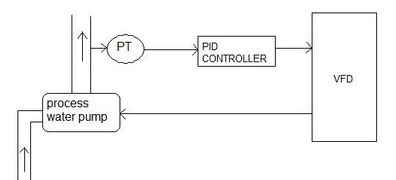The PID loop is used to maintain a process variable, such as speed. The desired speed, or setpoint & the actual speed values are input to a summation point. These two signals are opposite in polarity & yield a zero error or deviation whenever the desired speed equals the actual speed. If the two signals differ in value, the error signal will have a positive or negative value, depending on whether the actual speed is greater or less than the desired speed. This error signal is input to the PID controller. The terms proportional, integral, & derivative describe three basic mathematical functions then applied to the error signal. The PID output reacts to the error & outputs a frequency to try to reduce the error value to zero. The controller's job is to make the speed adjustments quickly, with a minimum of overshoot or oscillations. Tuning the PID controller involves gain & time adjustments designed to improve performance & result in a fast response with a minimum overshoot, allowing the motor to settle in quickly to the new speed. Some VFDs have a PID autotune function designed to ease the tuning process.

The PID controller can be used for process control. The connection of PID desired set value and PID real value of the application with the functions of the variable frequency drive enables process control without further components. In this way, VFD applications such as pressure, volume flow or speed control can be implemented easily.

The control deviation (difference between reference percentage and actual percentage is signaled to the PID controller. The PID controller adjusts the output frequency of the variable frequency drive such that the control deviation is minimized.

P controller: The output of the P controller is the variable frequency drive of the control deviation and the amplification and follows the control deviation linearly and without delay. A control deviation will be maintained.

I controller: The output of the I controller is the integral of the control deviation. The task of the I controller is to eliminate the control deviation. The integral time defines how fast the control deviation is compensated. If the I controller is set too dynamically (fast compensation of deviations), the variable frequency drive system may become unstable and vibrate. If the I controller is set too passively (slow compensation of deviations), the stationary error is not compensated sufficiently. For this reason, the integral portion must be adjusted plant-specifically.

D controller: The D controller assesses the change of the control deviation and calculates it change rate. This value is multiplied by the derivative time. The D controller responds to announced changes and causes a fast control behavior. The D controller can stabilize the VFD control circuit and reduce vibration. On the other hand, errors (e.g. interference voltages) are amplified.

Plz Calculate (2 + 3) =
(Your comment will show after approved.)

You may also like:

Category
Featured Articles
Variable frequency drive Rectifier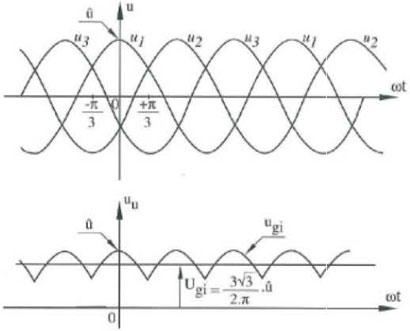To understand variable frequency drive (VFD) better, it's necessary to explain some of the main parts of the variable frequency ...
VFD controlled Induction motor ...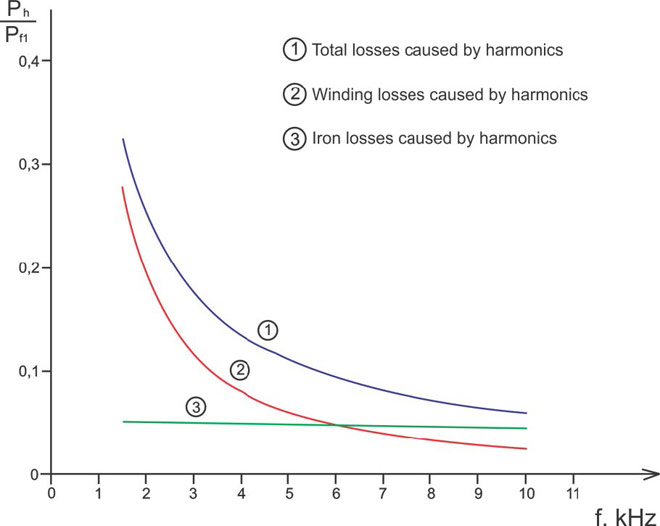This paper presents a procedure to measure the efficiency on an induction motor fed by a VFD by the all operation range to speed ...
VFD: Pulse Width Modulation (PWM)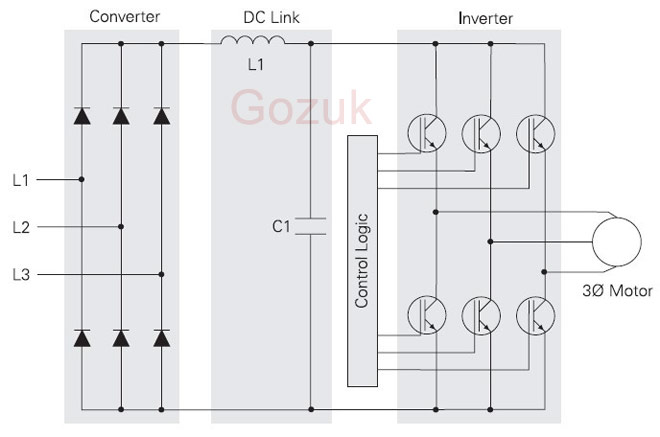Pulse Width Modulation (PWM) VFDs provide a more sinusoidal current output to control frequency and voltage supplied to an AC ...
Variable frequency drive Advantages & ... VFDs are good for variable speed, in a water pump this is used to maintain a steady pressure, they will smooth out variances in ...
Variable frequency drive Energy saving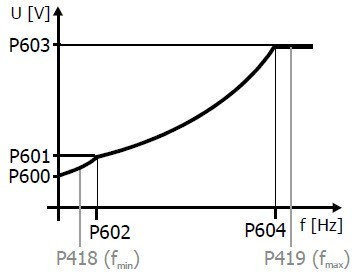Energy can be saved in a VFD by reducing the losses in the electric motor or by reducing the energy consumption of the variable ...VFD manufacturers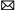buy@vfds.orgtech@vfds.org